## Introduction

In a previous post, I hinted at how to do multiple hypotheses testing, using the ψ-measure. It turns out to be much clearer just using the posterior probabilities. The ψ-measure has a nice intuitive feel for the two-hypothesis case, but becomes convoluted in the multiple hyptheses case. Further, when introducing the application of Bayes theorem for students, I have found it to be clearer to follow the following procedure. We first look at Bayes theorem directly, for N hypotheses:

﻿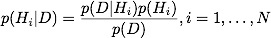We then calculate the numerator only, for every possible hypothesis:

﻿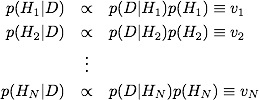calculate the sum of all of these values,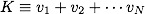￼and then normalize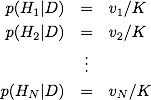## ﻿The Octopus, Again

From the Wikipedia article, we have the following data:, which gave us correct=12 out of N=14:

￼ ￼ ￼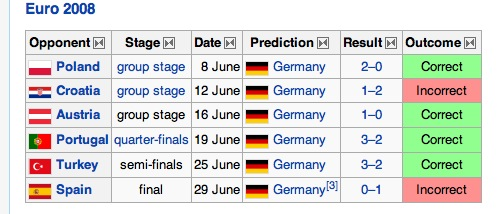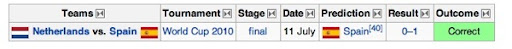The hypotheses that we consider are the following:

H = “Octopus is psychic, and can predict future (sports) events with 90% accuracy” R = “Octopus makes random choices” Y = “chooses flags with big yellow stripes 90% of the time” G = “chooses Germany 90% of the time”

Notice that both models Y and G, give us correct=12 for N=14 (if the “choosing Germany” chooses Spain in the Netherlands match, because of the similarity). The prior for the psychic octopus is, again, the very generous p(H) = 1/100. The two other non-random models should be more likely, before any data, so I take them to be p(Y)=p(G)=1/20. The random model, being the most likely, has the rest of the prior probability, p(R)=0.89.

Now we calculate the numerators:

﻿￼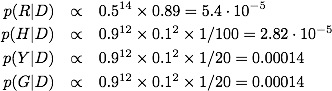Sum the values,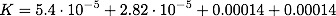and divide. achieving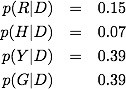Thus, the two flag models went from being rare compared to random to being much more likely than random, and certainly much more likely than psychic. Bayes theorem, properly applied, is a quantitative embodiment of Carl Sagan’s famous quote “extraordinary claims require extraordinary evidence”. It is not just that the evidence must be extraordinary (like 999 correct out of 1000), but the evidence must be extraordinary to address all of the, somewhat rare but possible, hypotheses that would come up as much more likely given the initial result. The process of science is to perform experiments to address these alternative hypotheses.

## Sunday, September 12, 2010

### God and Hawking

From the book “The Grand Design” By STEPHEN HAWKING And LEONARD MLODINOW

....
Newton believed that our strangely habitable solar system did not "arise out of chaos by the mere laws of nature." Instead, he maintained that the order in the universe was "created by God at first and conserved by him to this Day in the same state and condition."

....

The press is pitching this book as a denial of God, claiming that Hawking has said that God does not exist. The media never seem to get the nuances of logical thinking, and its consequences.

What Hawking and Mlodinow are doing is a modernization of an approach used by Laplace (1749-1827) (http://en.wikipedia.org/wiki/Pierre-Simon_Laplace).  He worked on many things, including the dynamics of the solar system.  When Newton (http://en.wikipedia.org/wiki/Isaac_Newton) published his laws of dynamics 100 years earlier, he demonstrated that the speeds of the planets could be derived from a simple law of gravity.  In this way, Newton connected the Earthly things with the "Heavenly" things.  However, it was unclear to Newton whether the orbits of the planets would remain constant (as his religious philosophy would state), or if they would be unstable, change, and possibly fly apart given enough time.  He posited that one of the roles of God would be to nudge the planets, here and there, to keep their orbits stable.

Laplace, performing his calculations more precisely than his predecessors, was able to determine that the orbits would in fact be stable, without any extra tinkering.  Napoleon, when presented with the work of Laplace, asked him: "M. Laplace, they tell me you have written this large book on the system of the universe, and have never even mentioned its Creator."  Laplace replied, "I had no need of that hypothesis."

He did not say that there was no God (although that is what he believed), but that the concept of God was not necessary to explain the things that he was explaining using physics.  This included the formation of the solar system from a compressing ball of gas (due to gravity), which then forms the Sun in the center and the planets orbiting around.  This is essentially the model still in use today!

What Hawking is doing is basically the same thing, but with the origin of the universe.  Essentially the current model allows for the possibility of many universes to simultaneously exist and that, like a lottery winner, our universe supports life.  It may seem that the universe is "fine-tuned" to support human life, and that this would support the notion of an intelligent designer, Hawking is making the argument that a designer is not needed with our current understanding.  Like a lottery winner stating that the odds of winning are astronomical, and yet they won, and then reasoning that there was some design in this choice even when there wasn't.  As long as you have enough people playing (or enough universes) you'll eventually observe the unlikely, and that unlikely winner will feel singled out.  Hawking argues that the lottery winner (the life on Earth), is arguing the same way when it invokes a designer when it doesn't need to.  Hawking doesn't state "God doesn't exist", because that statement cannot be proven, but he simply states that it is an unnecessary hypothesis for the understanding of the origin of the universe.

Of course, *specific* Gods can be disproven.  For example, it is clear from many lines of evidence that the Earth is more the 6000 years old and that there never was a global flood.  However, you cannot disprove the notion of a God that creates the universe and is then hands-off, like deists commonly believe.  It is completely untestable.  It is also unnecessary, according to Hawking.  This doesn't make it wrong, it is just unnecessary in the same way that we don't need to invoke the divine when understanding how an apple falls from a tree.

## Wednesday, September 1, 2010

### Why pseudoscientists like the chi-square test (and why it shouldn't be taught)

In a prior post I outlined how orthodox statistics can lead to the either-or logical fallacies common in pseudoscience, like astrology and ufo-ology.

In this post I focus on the &chi2 test, it's pathologies, and why it is so useful for a pseudoscientist. The example is lifted from E. T. Jaynes' book "Probability Theory"

The two problems with &chi2 are:

1. it violates your strong intuition in some simple cases
2. it can lead to different results with the exact same data, binned in a different way

Both of these properties are useful to the pseudoscientist.

## Intuition and Chi-square: The Three-sided coin

In each of this case we will have some data, and two models to compare which try to explain the data. Intuition strongly favors one, and &chi2 favors the other. One of my favorite problems is the three-sided coin: where the coin can fall heads, tails, or on the edge. Imagine we have two models for a relatively thick coin:

And we have the following data:

Which model are you more confident in? Model A of course! If we use the &psi-measure for goodness of fit with these two models, as defined in my prior post, then we have (remember: smaller &psi means more confident in the fit, just like smaller &chi2):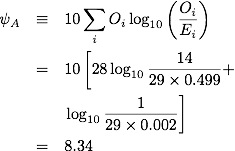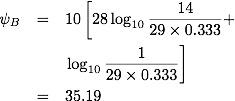with &psiB-&psiA=26.85 which makes model A more then 100 times more likely than model B (a &psi difference of 20 would be exactly 100 times). Perfectly reasonable. What about &chi2?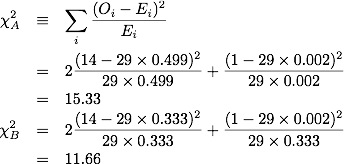which makes model B slightly preferable to model A! Amazing! Where is this coming from? Apparently it is coming from the somewhat rare event of an edge-landing. If our data had been instead

then we'd have

• &psiA=0.3
• &psiB=51.2

and

• &chi2A=0.093
• &chi2B=14.55

where now both measures agree that model A is superior.

 Why do pseudoscientists love the &chi2 test? Answer 1: Because all they need to do is wait for that inevitable, somewhat rare but still possible, data point and &chi2 yields a pathologically high value

## The &psi-measure and log-likelihood

To understand the other problem with the &chi2 test we need to understand what the &psi-measure is doing. As above, imagine we have a set of observations Oi. We define the total number of observed points and the relative frequency of each observation,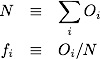The maximum likelihood solution for the probabilities of observing Oi for each class, i, is just the relative frequency of each observation. This is the "just-so" solution, where we estimate the probability of seeing 14 heads in 29 flips as p=14/29. This "just-so" solution will have the closest match, and the highest likelihood (by definition). If we have a model which specifies a different set of probabilities for each class, then it's likelihood is simply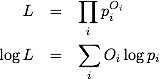The &psi measure can be rewritten as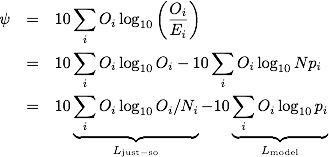So you can think of the &psi-measure as comparing a model with the "just-so" solution (which has maximum likelihood). Further, subtracting one value of &psi with another (for different models) performs the log-likelihood ratio between the models. A proper analysis should include prior information, which can be done almost as easily.

## An almost equivalent problem

Imagine that we have a coin with 6 faces, and we are comparing the following models:

• Model A: p = [0.499/2, 0.499/2, 0.499/2, 0.499/2, 0.002/2,0.002/2]
• Model B: p = [1/6,1/6,1/6,1/6,1/6,1/6]

And we have the following data:

• N=29: O=[7,7,7,7,0,1]

where I have listed the probabilities and the outcomes for each face. Notice that, grouping them together in pairs we retrieve the same as the first example. Thus when comparing the two models, with this equivalent problem, we should get the same value. Because the size of the problem changed, the individual &psi values will be different (larger) because there are more terms in the "just-so" solution. However, the difference between the models should be the same. The results are:

• &psiA=11.35 (old value 8.34)
• &psiB=38.2 (old value 35.19)

with &psiB-&psiA=26.85 (old value 26.85...the same!), and

• &chi2A=32.6 (old value 15.33)
• &chi2B=11.76 (old value 11.66)

The &chi2 for one of the models (Model A) has been inflated quite a lot relative to the other model. This means that, depending on how you bin the data, you can make whichever model that you are looking at more or less significantly different, without changing the data at all.

 Why do pseudoscientists love the &chi2 test? Answer 2: Because all they need to do is bin their data in different ways to affect the level of significance of their model over the model to which they are comparing

## Still taught?

So, why is the &chi2 test still taught? I don't know. It has pathological behavior in simple systems, where somewhat rare events artificially inflate its value, and it can be easily used to prop up an unreasonable model simply by rearranging the data. Why not teach something, like the &psi-measure, which is grounded theoretically in the likelihood principle and does not have such pathological behavior? If you prefer to use the log-likelihood instead, then that would be fine (and equivalent).

I think it is about time to purge the &chi2 test from our textbooks, and replace it with something correct.